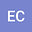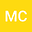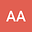•••Much of the water that once flowed on the surface of Mars was lost to space long ago, and the total amount lost remains unknown. Clues to the amount lost can be found by studying hydrogen (H) and its isotope deuterium (D), both of which are produced when atmospheric water molecules H$_2$O and HDO dissociate. The freed H and D atoms then escape to space at different rates due to their different masses, leaving an enhanced D/H ratio. The rate of change of D/H is referred to as the fractionation factor $f$. Both the D/H ratio and $f$ are necessary to estimate water loss; thus, if we can constrain the range of $f$, we will be able to estimate water loss more accurately. In this study, we use a 1D photochemical model of the Martian atmosphere to determine how $f$ depends on assumed temperature and water vapor profiles. We find that for most Martian atmospheric conditions, $f$ varies between $10^{-1}$ and $10^{-5}$; for the standard Martian atmosphere, $f=0.002$ for thermal escape processes, and $f\approxeq0.06$ when both thermal and non-thermal escape are considered. Using these results, we estimate that Mars has lost at minimum 66-123 m GEL of water. Our results demonstrate that the value of $f$ is almost completely controlled by the amount of non-thermal escape of D, and that photochemical modeling studies that include fractionation must thus model both neutral and ion processes throughout the atmosphere.